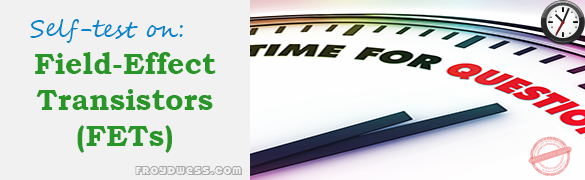# Floyd Self-test in Field-Effect Transistors (FETs)

(Last Updated On: December 8, 2017)This is the Self-test in Chapter 8: Field-Effect Transistors (FETs) from the book Electronic Devices Conventional Current Version, 9th edition by Thomas L. Floyd. If you are looking for a reviewer in Electronics Engineering this will definitely help you before taking the Board Exam.

### Floyd Self-test Chapter 8 Topic Outline

• Floyd Self-test in Field-Effect Transistors (FETs)
• Floyd Self-test in JFET
• Floyd Self-test in JFET Characteristics and Parameters
• Floyd Self-test in JFET Biasing
• Floyd Self-test in The Ohmic Region
• Floyd Self-test in MOSFET
• Floyd Self-test in MOSFET Characteristics and Parameters
• Floyd Self-test in MOSFET Biasing
• Floyd Self-test in IGBT

### Start Practice Exam Test Questions

Choose the letter of the best answer in each questions.

1. The JFET is

• (a) a unipolar device
• (b) a voltage-controlled device
• (c) a current-controlled device
• (d) answers (a) and (c)
• (e) answers (a) and (b)

2. The channel of a JFET is between the

• (a) gate and drain
• (b) drain and source
• (c) gate and source
• (d) input and output

3. A JFET always operates with

• (a) the gate-to-source pn junction reverse-biased
• (b) the gate-to-source pn junction forward-biased
• (c) the drain connected to ground
• (d) the gate connected to the source

4. For VGS = 0 V, the drain current becomes constant when VDS exceeds

• (a) cutoff
• (b) VDD
• (c) VP
• (d) 0 V

5. The constant-current region of a FET lies between

• (a) cutoff and saturation
• (b) cutoff and pinch-off
• (c) 0 and IDSS
• (d) pinch-off and breakdown

6. IDSS is

• (a) the drain current with the source shorted
• (b) the drain current at cutoff
• (c) the maximum possible drain current
• (d) the midpoint drain current

7. Drain current in the constant-current region increases when

• (a) the gate-to-source bias voltage decreases
• (b) the gate-to-source bias voltage increases
• (c) the drain-to-source voltage increases
• (d) the drain-to-source voltage decreases

8. In a certain FET circuit, VGS = 0 V, VDD = 15 V, IDSS = 15 mA, and RD = 470Ω. If RD is decreased to 330Ω, IDSS is

• (a) 19.5 mA
• (b) 10.5 mA
• (c) 15 mA
• (d) 1 mA

9. At cutoff, the JFET channel is

• (a) at its widest point
• (b) completely closed by the depletion region
• (c) extremely narrow
• (d) reverse-biased

10. A certain JFET datasheet gives The pinch-off voltage, VP,

• (a) cannot be determined
• (b) is – 4V
• (c) depends on VGS
• (d) is – 4 V

11. The JFET in Question 10

• (a) is an n channel
• (b) is a p channel
• (c) can be either

12. For a certain JFET, IGSS = 10 nA at VGS =10 V. The input resistance is

• (a) 100MΩ
• (b) 1MΩ
• (c) 1000 MΩ
• (d) 1000mΩ

13. For a certain p-channel JFET, VGS(off ) = 8 V. The value of VGS for an approximate midpoint bias is

• (a) 4 V
• (b) 0 V
• (c) 1.25 V
• (d) 2.34 V

14. In a self-biased JFET, the gate is at

• (a) a positive voltage
• (b) 0 V
• (c) a negative voltage
• (d) ground

15. The drain-to-source resistance in the ohmic region depends on

• (a) VGS
• (b) the Q-point values
• (c) the slope of the curve at the Q-point
• (d) all of these

16. To be used as a variable resistor, a JFET must be

• (a) an n-channel device
• (b) a p-channel device
• (c) biased in the ohmic region
• (d) biased in saturation

17. When a JFET is biased at the origin, the ac channel resistance is determined by

• (a) the Q-point values
• (b) VGS
• (c) the transconductance
• (d) answers (b) and (c)

18. A MOSFET differs from a JFET mainly because

• (a) of the power rating
• (b) the MOSFET has two gates
• (c) the JFET has a pn junction
• (d) MOSFETs do not have a physical channel

19. A D-MOSFET operates in

• (a) the depletion mode only
• (b) the enhancement mode only
• (c) the ohmic region only
• (d) both the depletion and enhancement modes

20. An n-channel D-MOSFET with a positive VGS is operating in

• (a) the depletion mode
• (b) the enhancement mode
• (c) cutoff
• (d) saturation

21. A certain p-channel E-MOSFET has a If VGS = 0 V, the drain current is

• (a) 0 A
• (b) ID(on)
• (c) maximum
• (d) IDSS

22. In an E-MOSFET, there is no drain current until VGS

• (a) reaches VGS(th)
• (b) is positive
• (c) is negative
• (d) equals 0 V

23. All MOS devices are subject to damage from

• (a) excessive heat
• (b) electrostatic discharge
• (c) excessive voltage
• (d) all of these

24. A certain D-MOSFET is biased at VGS = 0 V. Its datasheet specifies IDSS= 20 mA and the value of the drain current

• (a) is 0 A
• (b) cannot be determined
• (c) is 20 mA

25. An IGBT is generally used in

• (a) low-power applications
• (b) rf applications
• (c) high-voltage applications
• (d) low-current applications

Rate this: